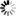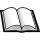Dr. S. R. Lasker Library Online Catalogue

Normal view

# Algorithms for statistical signal processing / John G. Proakis ... [et al.].

Material type:TextLanguage: English Publication details: c2002. Description: xii, 564 p. : ill. ; 24 cmISBN: 8178085739; 9780130622198; 0130622192DDC classification: 621.3822 LOC classification: TK5102.9 | .A43 2002Online resources: WorldCat details
Contents:
TOC Characterization of Signals -- Characterization of Linear Time-Invariant Systems -- Sampling of Signals -- Linear Filtering Methods Based on the DFT -- The Cepstrum -- Algorithms for Convolution and Dft -- Modulo Polynomials -- Circular Convolution as Polynomial Multiplication mod u[superscript N]--1 -- A Continued Fraction of Polynomials -- Chinese Remainder Theorem for Polynomials -- Algorithms for Short Circular Convolutions -- How We Count Multiplications -- Cyclotomic Polynomials -- Elementary Number Theory -- Convolution Length and Dimension -- The DFT as a Circular Convolution -- Winograd's DFT Algorithm -- Number-Theoretic Analogy of DFT -- Number-Theoretic Transform -- Split-Radix FFT -- Autogen Technique -- Linear Prediction and Optimum Linear Filters -- Innovations Representation of a Stationary Random Process -- Forward and Backward Linear Prediction -- Solution of the Normal Equations -- Properties of the Linear Prediction-Error Filters -- AR Lattice and ARMA Lattice-Ladder Filters -- Wiener Filters for Filtering and Prediction -- Least-Squares Methods for System Modeling and Filter Design -- System Modeling and Identification -- Least-Squares Filter Design for Prediction and Deconvolution -- Solution of Least-Squares Estimation Problems -- Adaptive Filters -- Applications of Adaptive Filters -- Adaptive Direct-Form FIR Filters -- Adaptive Lattice-Ladder Filters -- Recursive Least-Squares Algorithms for Array Signal Processing -- QR Decomposition for Least-Squares Estimation.
List(s) this item appears in: Signal and System
Tags from this library: No tags from this library for this title.
Star ratingsAverage rating: 0.0 (0 votes)
Holdings
Item type Current library Collection Call number Copy number Status Date due Barcode Item holdsText
Reserve Section
Non-fiction 621.3822 ALG 2002 (Browse shelf(Opens below)) C-1 Not For Loan 13142Text
Circulation Section
Non-fiction 621.3822 ALG 2002 (Browse shelf(Opens below)) C-2 Available 13143Text
Circulation Section
Non-fiction 621.3822 ALG 2002 (Browse shelf(Opens below)) C-3 Available 13144
Total holds: 0

Online version:
Algorithms for statistical signal processing.
Upper Saddle River, N.J. : Prentice Hall, c2002
(OCoLC)606578408

Includes bibliographical references (p. 542-558) and index.

TOC Characterization of Signals --
Characterization of Linear Time-Invariant Systems --
Sampling of Signals --
Linear Filtering Methods Based on the DFT --
The Cepstrum --
Algorithms for Convolution and Dft --
Modulo Polynomials --
Circular Convolution as Polynomial Multiplication mod u[superscript N]--1 --
A Continued Fraction of Polynomials --
Chinese Remainder Theorem for Polynomials --
Algorithms for Short Circular Convolutions --
How We Count Multiplications --
Cyclotomic Polynomials --
Elementary Number Theory --
Convolution Length and Dimension --
The DFT as a Circular Convolution --
Number-Theoretic Analogy of DFT --
Number-Theoretic Transform --
Autogen Technique --
Linear Prediction and Optimum Linear Filters --
Innovations Representation of a Stationary Random Process --
Forward and Backward Linear Prediction --
Solution of the Normal Equations --
Properties of the Linear Prediction-Error Filters --
AR Lattice and ARMA Lattice-Ladder Filters --
Wiener Filters for Filtering and Prediction --
Least-Squares Methods for System Modeling and Filter Design --
System Modeling and Identification --
Least-Squares Filter Design for Prediction and Deconvolution --
Solution of Least-Squares Estimation Problems --
Recursive Least-Squares Algorithms for Array Signal Processing --
QR Decomposition for Least-Squares Estimation.

EEE

Saifun Momota

There are no comments on this title.